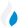•Trade Pi
•Trade
•Exchange
•Trade Pi
•Trade
•Exchange
\$1.1T
Total marketcap
\$66.55B
Total volume
40.09%
BTC dominance
•Trade Pi
•Trade
•Exchange

# QNT to MATIC Exchange Rate - 1 Quant in Polygon

116.34515219788
• BTC 0.006
• ETH 0.08
Vol [24h]
\$9,845.25

## qnt to matic converter

Exchange Pair Price 24h volume
Uniswap (v3) QNT/MATIC \$138.27 \$9.85K

## QNT/MATIC Exchange Rate Overview

Name Ticker Price % 24h 24h high 24h low 24h volume
Quant qnt \$143.05 -1.9979% \$146.19 \$142.87 \$18.07M
Polygon matic \$1.27 1.8692% \$1.28 \$1.22 \$616.6M

Selling 1 QNT you get 116.34515219788 Polygon matic.

Quant Sep 11, 2021 had the highest price, at that time trading at its all-time high of \$427.42.

513 days have passed since then, and now the price is 32.52% of the maximum.

Based on the table data, the QNT vs MATIC exchange volume is \$9,845.25.

Using the calculator/converter on this page, you can make the necessary calculations with a pair of Quant/Polygon.

## Q&A

### What is the current QNT to MATIC exchange rate?

Right now, the QNT/MATIC exchange rate is 116.34515219788.

### What has been the Quant to Polygon trading volume in the last 24 hours?

Relying on the table data, the Quant to Polygon exchange volume is \$9,845.25.

### How can I calculate the amount of MATIC? / How do I convert my Quant to Polygon?

You can calculate/convert MATIC from Quant to Polygon converter. Also, you can select other currencies from the drop-down list.

## QNT to MATIC Сonversion Table

QNT MATIC
0.003 QNT = 0.3 MATIC
0.007 QNT = 0.8 MATIC
0.01 QNT = 1.1 MATIC
0.02 QNT = 2.3 MATIC
0.03 QNT = 3.4 MATIC
0.07 QNT = 8.1 MATIC
0.1 QNT = 11.6 MATIC
0.3 QNT = 34.9 MATIC
0.7 QNT = 81.4 MATIC
7 QNT = 814.4 MATIC
80 QNT = 9,307.6 MATIC
800 QNT = 93,076.1 MATIC
8000 QNT = 930,761.2 MATIC